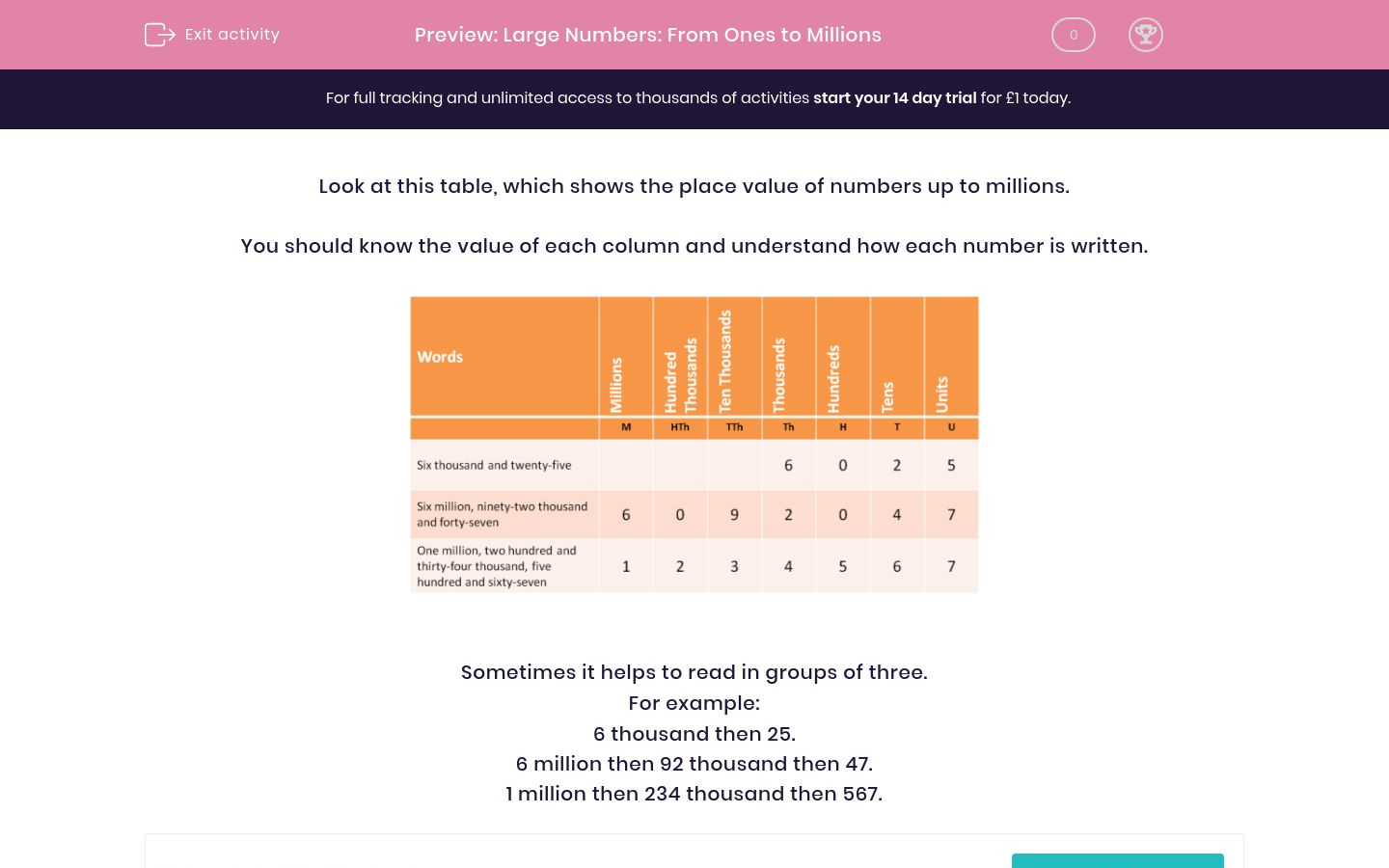# Large Numbers: From Ones to Millions

In this worksheet, students read and write numbers from 1 to 10 000 000.Key stage:  KS 2

Curriculum topic:   Maths and Numerical Reasoning

Curriculum subtopic:   Place Value

Difficulty level:### QUESTION 1 of 10

Look at this table, which shows the place value of numbers up to millions.

You should know the value of each column and understand how each number is written.Sometimes it helps to read in groups of three.

For example:

6 thousand then 25.

6 million then 92 thousand then 47.

1 million then 234 thousand then 567.Use the columns above to write the following number using digits.

Five million, three hundred and fifty-two thousand and ninety-sixUse the columns above to write the following number using digits.

Three million, fifty-two thousand and ninety-sixUse the columns above to write the following number using digits.

Fifty-two thousand, one hundred and thirty-sixUse the columns above to write the following number using digits.

Six million, fifty-three thousand and sixUse the columns above to write the following number using digits.

Five million, six hundred and fifty-three thousand, seven hundred and ninety-sixUse the columns above to read the following number.

40 089

four million and eighty-nine

forty thousand and eighty-nine

forty-eight thousand and nineUse the columns above to read the following number.

4 214 089

four million, two hundred and fourteen, and eighty-nine

four million, two hundred and fourteen thousand, and eighty-nine

four million, two hundred and fourteen hundred, and eighty-nineUse the columns above to read the following number.

404 809

four million forty-eight thousand and nine

forty thousand, four thousand and eighty-nine

four hundred and four thousand, eight hundred and nineUse the columns above to read the following number.

444 040

four million, four thousand and forty

four million, four hundred and forty thousand

four hundred and forty-four thousand and fortyUse the columns above to read the following number.

4 404 400

four million, four hundred and four thousand, four hundred

four million, four hundred and forty-four thousand

four hundred million and four thousand and forty

• Question 1Use the columns above to write the following number using digits.

Five million, three hundred and fifty-two thousand and ninety-six

5352096
5 352 096
EDDIE SAYS
Nothing in the hundreds column.
• Question 2Use the columns above to write the following number using digits.

Three million, fifty-two thousand and ninety-six

3052096
3 052 096
EDDIE SAYS
Nothing in the hundred thousands column or the hundreds column.
• Question 3Use the columns above to write the following number using digits.

Fifty-two thousand, one hundred and thirty-six

52136
52 136
EDDIE SAYS
Just enter the digits in the columns.
• Question 4Use the columns above to write the following number using digits.

Six million, fifty-three thousand and six

6053006
6 053 006
EDDIE SAYS
Nothing in the hundred thousands column or in the hundreds or tens.
• Question 5Use the columns above to write the following number using digits.

Five million, six hundred and fifty-three thousand, seven hundred and ninety-six

5653796
5 653 796
EDDIE SAYS
Just enter the numbers in the columns.
• Question 6Use the columns above to read the following number.

40 089

forty thousand and eighty-nine
EDDIE SAYS
40 thousand, no hundreds and 89.
• Question 7Use the columns above to read the following number.

4 214 089

four million, two hundred and fourteen thousand, and eighty-nine
EDDIE SAYS
Read in groups of three: 4 million then 214 thousand then 89.
• Question 8Use the columns above to read the following number.

404 809

four hundred and four thousand, eight hundred and nine
EDDIE SAYS
Read in groups of three: 404 thousand then 809.
• Question 9Use the columns above to read the following number.

444 040

four hundred and forty-four thousand and forty
EDDIE SAYS
Read in groups of three: 444 thousand then 40.
• Question 10Use the columns above to read the following number.

4 404 400

four million, four hundred and four thousand, four hundred
EDDIE SAYS
Read in groups of three: 4 million then 404 thousand then 400.
---- OR ----

Sign up for a £1 trial so you can track and measure your child's progress on this activity.

### What is EdPlace?

We're your National Curriculum aligned online education content provider helping each child succeed in English, maths and science from year 1 to GCSE. With an EdPlace account you’ll be able to track and measure progress, helping each child achieve their best. We build confidence and attainment by personalising each child’s learning at a level that suits them.

Get started